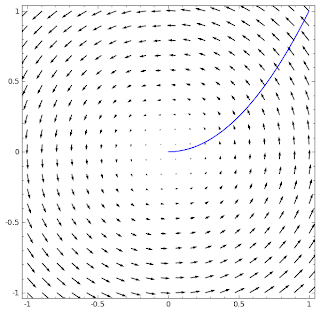## Vector field examplesage: p1 = plot_vector_field((-y, x), (x, -1, 1), (y, -1, 1))
sage: p2 =implicit_plot(x^2-y, (x, 0, 1), (y, -1, 1))
sage: show(p1+p2)

Remember that in physics work is $W = Fr$, force times distance. When these two are not in the same direction, you need to calculate the component of force along the direction of movement. In the language of matrix algebra, $W = F \cdot r = |F| |r| \cos \theta$. Now suppose we have a force field (see the figure): \begin{align*} F &= \begin{pmatrix} -y \\ x \end{pmatrix} \end{align*} and a object moved along $y = x^2$ with $x$ ranging from 0 to 1. The question is how much work is done on the object? Solution 1: Parameterize, \begin{align*} \begin{pmatrix} x \\ y \end{pmatrix} &= \begin{pmatrix} t \\ t^2 \end{pmatrix} \\ \\ dr = (dr/dt) dt &= \begin{pmatrix} 1 \\ 2t \end{pmatrix} dt \\ \\ F = \begin{pmatrix} -y \\ x \end{pmatrix} &= \begin{pmatrix} -t^2 \\ t \end{pmatrix} \\ \\ F \cdot dr &= t^2 \; dt \\ \\ W = \int_{Curve} F\cdot dr &= \int_0^1 t^2 dt \\ &= 1/3 \end{align*} Solution 2: Convert to single variable. \begin{align*} F \cdot dr &= \begin{pmatrix} -y \\ x \end{pmatrix} \cdot \begin{pmatrix} dx \\ dy \end{pmatrix} \\ &= -ydx + xdy \\ &= -x^2 dx + x (2x dx) \\ &= x^2 dx \\\\ W = \int_{Curve} F\cdot dr &= \int_0^1 x^2 dx \\ &= 1/3 \end{align*}